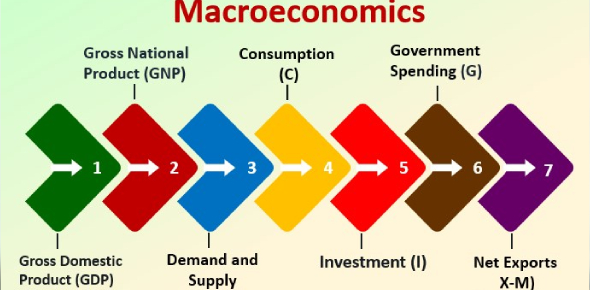# A Macroeconomics Practice Quiz!

9 Questions | Total Attempts: 19867SettingsMacroeconomics is the branch of economics that deals with the performance, structure, behavior, and decision-making of an economy as a whole. Do you need some practice for an upcoming assignment or test? Do you wanna test your knowledge on it? Take the Quiz!

• 1.
Diffrence between Real and Nominal GDP is:
• A.

Measured by excluding some of the sectors

• B.

That real GDP is always smaller than Nominal GDP.

• C.

Change in price level from base year to current year.

• D.

None of the above.

• 2.
GDP is the market value of all the final goods:
• A.

Produced domestically.

• B.

Produced by domestic factors of production.

• C.

Produced by all factors of production.

• D.

All of the above.

• 3.
When comparing a nation's economic position with another, one should see its:
• A.

GDP

• B.

Per Capita GDP.

• C.

Currency in circulation.

• D.

None of the above.

• 4.
Verticle intercept of consumption function indicates:
• A.

Average propensity to consume.

• B.

Consumption at zero level of income.

• C.

Both of the above.

• D.

None of the above.

• 5.
In consumption function, c = a+by
• A.

B is intercept

• B.

Slope of the function

• C.

MPC

• D.

a & C

• 6.
In Keynesian Framework, Income is measured along:
• A.

45 degree line

• B.

Verticle axis.

• C.

Horizontal axis.

• D.

All are correct.

• 7.
If investment is exogenous to the Income determination model it can be shown as
• A.

Verticle line.

• B.

Horizontal line.

• C.

All of the above.

• D.

None of the above.

• 8.
In closed economy GDP equals
• A.

C+I+X-N

• B.

C+I

• C.

C+I+G

• D.

All of the above.

• 9.
In the model Y=C+I+G and C=a+by, Where b=0.8, the expenditure multiplier is
• A.

1

• B.

4

• C.

5

• D.

None of the above

Related TopicsBack to top